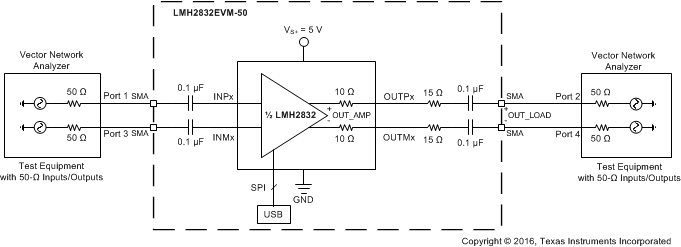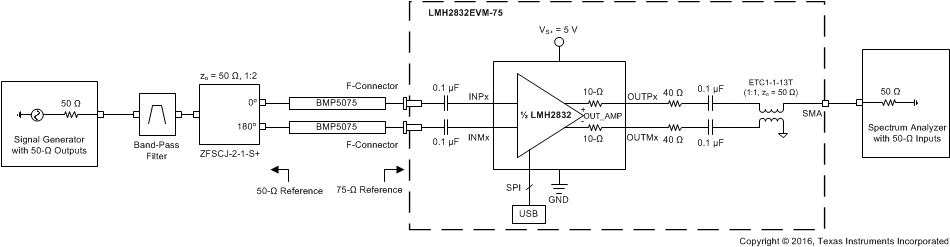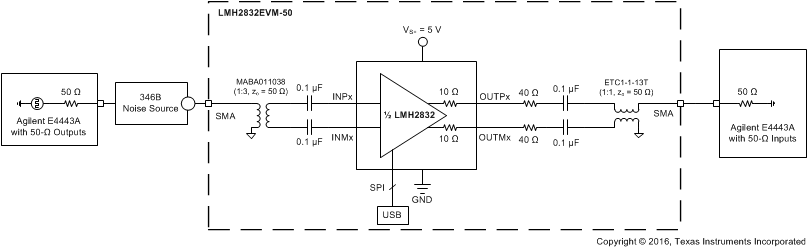SBOS709A July   2016  – July 2016

PRODUCTION DATA.

1. Features
2. Applications
3. Description
4. Revision History
5. Device Comparison Table
6. Pin Configuration and Functions
7. Specifications
8. Parameter Measurement Information
9. Detailed Description
1. 9.1 Overview
2. 9.2 Functional Block Diagram
3. 9.3 Feature Description
4. 9.4 Device Functional Modes
5. 9.5 Programming
6. 9.6 Register Maps
10. 10Application and Implementation
1. 10.1 Application Information
2. 10.2 Typical Applications
1. 10.2.1 DOCSIS 3.X Driver
3. 10.3 Do's and Don'ts
11. 11Power Supply Recommendations
12. 12Layout
13. 13Device and Documentation Support
14. 14Mechanical, Packaging, and Orderable Information

• RHA|40
• RHA|40

## 8 Parameter Measurement Information

### 8.1 Setup Diagrams

Figure 40 to Figure 43 illustrate various test setup diagrams using the LMH2832 evaluation module (EVM).Figure 40. Frequency Response Differential Test SetupFigure 41. Single-Tone Harmonic Distortion Test SetupFigure 42. Two-Tone Linearity Test Setup (OIP3, OIP2)Figure 43. Noise Figure Test Setup

### 8.2 ATE Testing and DC Measurements

All production testing and dc parameters are measured on automated test equipment (ATE) capable of dc measurements only. Some measurements (such as voltage gain) are referenced to the output of the internal amplifier and do not include losses attributed to the on-chip output resistors. The Electrical Characteristics values specify these conditions. When the measurement is referred to the amplifier output, the output resistors are not included in the measurement. If the measurement is referred to the device pins, then the output resistor loss is included in the measurement.

### 8.3 Frequency Response

This test is done by running an S-parameter sweep on a 4-port differential network analyzer using the standard EVM with no baluns; see Figure 40. The inputs and outputs of the EVM are connected to the network analyzer using 75-Ω coaxial cables with the input ports set to a characteristic impedance of 75 Ω, and the output ports set to a characteristic impedance of 50 Ω.

The frequency response test with capacitive load is done by soldering the capacitor across the LMH2832 output pins. In this configuration, the on-chip, 10-Ω resistors on each output leg isolate the capacitive load from the amplifier output pins.

### 8.4 Distortion

The standard EVM is used for measuring both the single-tone harmonic distortion and two-tone intermodulation distortion; see Figure 41 and Figure 42, respectively. The distortion is measured with differential input signals to the LMH2832. In order to interface with 50-Ω, single-ended test equipment, 50-Ω to 75-Ω impedance matching pads followed by external baluns (1:2, zo = 75 Ω) are required between the EVM output ports and the test equipment. These baluns are used to combine two single tones in the two-tone test plots as well as to convert the single-ended input to differential output for harmonic distortion tests. The use of 6-dB attenuator pads on both the inputs and outputs is recommended to provide a balanced match between the external balun and the EVM.

### 8.5 Noise Figure

This test is done by matching the input of the LMH2832 to a 50-Ω noise source using a 50-Ω to 75-Ω impedance transformation pad followed by a 1:2 balun (Figure 43), with the noise figure being referred to the input impedance (RS = 150 Ω). As noted in Figure 43, a Keysight Technologies™ E4443A with NF features is used for the testing.

### 8.6 Pulse Response, Slew Rate, and Overdrive Recovery

For time-domain measurements, the standard EVM is driven through an impedance transformation pad and a balun again to convert a single-ended output from the test equipment to the differential inputs of the LMH2832. The differential outputs are directly connected to the oscilloscope inputs, with the differential signal response calculated using trace math from the two separate oscilloscope inputs.

### 8.7 Power-Down

The standard EVM is used for this test by completely removing the shorting block on jumper JPD. A high-speed, 50-Ω pulse generator is used to drive the PDx pin that toggles the output signal on or off depending upon the PDx pin voltage.

### 8.8 Crosstalk, Gain Matching, and Phase Matching

The standard EVM is used for these tests with both channels enabled. For gain and phase matching, the responses of both channels are measured on a network analyzer and the gain and phase values are compared. For crosstalk, a single channel is driven with a signal on the network analyzer when the other channel is measured.

### 8.9 Output Measurement Reference Points

The LMH2832 has two on-chip, 10-Ω output resistors on each channel. When matching the output to a 100-Ω load, the evaluation module (EVM) uses an external 40-Ω resistor on each output leg to complete the output matching. The inclusion of on-chip output resistors creates two potential reference points for measuring the output voltage. The first reference point is at the internal amplifier output (OUT_AMP), and the second reference point is at the externally-matched 100-Ω load (OUT_LOAD). The measurements in the Electrical Characteristics table and in the Typical Characteristics section are referred to the (OUT_AMP) reference point unless otherwise specified. The conversion between reference points is a straightforward correction of 3 dB for power and 6 dB for voltage, as shown in Equation 1 and Equation 2. The measurements are referenced to OUT_AMP when not specified.

Equation 1. VOUT_LOAD = (VOUT_AMP – 6 dB)
Equation 2. POUT_LOAD = (POUT_AMP – 3 dB)